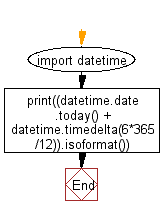﻿ Python: Calculates the date six months from the current date - w3resource# Python: Calculates the date six months from the current date

## Python Datetime: Exercise-26 with Solution

Write a Python program calculates the date six months from the current date using the datetime module.

Sample Solution:

Python Code:

``````import datetime
print((datetime.date.today() + datetime.timedelta(6*365/12)).isoformat())
```
```

Sample Output:

```2017-11-04
```

Flowchart:## Visualize Python code execution:

The following tool visualize what the computer is doing step-by-step as it executes the said program:

Python Code Editor:

What is the difficulty level of this exercise?

Test your Python skills with w3resource's quiz

﻿

## Python: Tips of the Day

Free up Memory:

• Manual garbage collection can be performed on timely or event based mechanism.
```import gc
collected_objects = gc.collect()
```